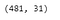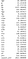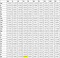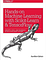# NBA Data Analysis Using Python & Machine Learning

Explore NBA Basketball Data Using KMeans Clustering

In this article I will show you how to explore data and use the unsupervised machine learning algorithm called KMeans to cluster / group NBA players. The code will explore the NBA players from 2013–2014 basketball season and use KMeans to group them in clusters to show which players are most similar.

K-Means is one of the most popular “clustering” algorithms. K-means stores ‘k’ centroids that it uses to define clusters. A point is considered to be in a particular cluster if it is closer to that cluster’s centroid than any other centroid. K-Means finds the best centroids by alternating between (1) assigning data points to clusters based on the current centroids (2) chosing centroids (points which are the center of a cluster) based on the current assignment of data points to clusters. — Chris Piech

The KMeans algorithm will categorize the items into k groups of similarity. To calculate that similarity, we will use the euclidean distance as measurement.

The K-Means algorithm works as follows:

1. First we initialize k points, called means, randomly.

The “points” mentioned above are called means, because they hold the mean values of the items categorized in it. -geeksforgeeks

If you prefer not to read this article and would like a video representation of it, you can check out the YouTube video below. It goes through everything in this article with a little more detail, and will help make it easy for you to start programming your own Machine Learning model in Python. Or you can use both (this article and video) as supplementary materials for learning about Machine Learning !

# Start Programming & Exploring

First thing I like to do when creating my programs is to describe what that program is doing, so first I will write comments explaining what the program is doing.

`# This code explores the NBA players from 2013 - 2014 basketball season, and uses # a machine learning algorithm called kMeans to group them in clusters, this will # show which players are most similar`

Import some packages that will be used regularly throughout the program. Pandas for dataframes, seaborn for correlations and heat maps, and matplotlib.pyplot for plots.

`#import the dependenciesimport pandas as pdimport seaborn as snsimport matplotlib.pyplot as plt`

Load the NBA 2013–2014 Data. Store the data into a data frame called ‘nba’, and print the first 7 rows of data / players. Now I can see data on players like Steven Adams, and LaMarcus Aldridge.

`#load the data #from google.colab import files #Only use for Google Colab#uploaded = files.upload()      #Only use for Google Colabnba = pd.read_csv('nba_2013.csv')# the nba_2013.csv data nba.head(7)# Print the first 7 rows of data or first 7 players`

See how many players there were this season by getting the number of rows, since every row of data contains information on a single player, and get the number columns or features of each player. I see that there are 481 players for this season and 31 features of each player.

`#Get the number of rows and columns (481 rows or players , and 31 columns containing data on the players)nba.shape`481 players and 31 features of each player in the data set

Find the average or mean for each numeric column / feature in the data set. By using the mean method, I can see that the average age of an NBA player for that season is 26.5, and I can expect the average player to get about 516 points (pts) in a season, 24 blocks (blk), 39 steals (stl)and 113 assists (ast). Which is pretty cool. If you do not know what these columns mean here is a glossary, and another one here. What’s interesting is that LaMarcus made about 4 times as many field goals (fg) as the average player.

`nba.mean()`The mean/average of the statistics for players during the 2013–2014 NBA season

I only want to look at the average field goals (fg) made for the season. When looking at the mean of only one specific column I get back a number with a few more numbers after the decimal. In this case it’s 192.88149688149687, about the same as I saw before when I first got the mean of all of the columns.

`nba.loc[:,"fg"].mean()`

Explore the data even more by creating pairwise scatter plots, this will allow me to see how different columns correlate to others. I will only compare assists (ast), field goals(fg), and total rebounds(trb). I can see correlations but I am not sure how positive or negative that correlation is. I might need to create a heat map to visual this better.

`sns.pairplot(nba[["ast", "fg", "trb"]])plt.show()`

To get a better visualization I will create a heat map of the columns assists (ast), field goals(fg), and total rebounds(trb). Now I can see how positive and negative the correlations are.

`correlation = nba[["ast", "fg", "trb"]].corr()sns.heatmap(correlation, annot=True)`

Now I want to make 5 clusters of players using the machine learning model called KMeans to show which players are most similar. First I need to create the model and store it in a variable called kmeans_model. Second I need to clean the data, so I will get only the numeric data and drop any columns with missing data and store it in a variable called good_columns. Then I need to train the model on the new data ‘good_columns’. Once it is done training I will get the labels from the model and store it in a variable called labels and print the labels for each row of data / player to the screen. The labels will be classifiers from 0 to 4, since I want 5 clusters.

`from sklearn.cluster import KMeanskmeans_model = KMeans(n_clusters=5, random_state=1)good_columns = nba._get_numeric_data().dropna(axis=1)kmeans_model.fit(good_columns)labels = kmeans_model.labels_labels`

Now I want a visualization of this data, so I will plot the players by cluster. This can be achieved by using the Principal Component Analysis (PCA) method to to make the data 2 dimensions, then plot it and shade each point according to the cluster association.

`from sklearn.decomposition import PCApca_2 = PCA(2)plot_columns = pca_2.fit_transform(good_columns)plt.scatter(x=plot_columns[:,0], y=plot_columns[:,1], c=labels)plt.show()`

To see the coordinates / points of each player.

`plot_columns`

I am interested to see if LeBron James and Kevin Durant (two great basketball players) are in the same cluster or not, given these 5 clusters. So First I need to find the players from the data used to cluster the players which is stored in the variable ‘good_columns’, and I want to print their data to the screen. When printing their data to the screen I can see the number of steels (stl) for the NBA season for both players is above average as well as the field goals (fg) made. This information backs the fact that these two are great players above average.

`# Find player LeBronLeBron = good_columns.loc[ nba['player'] == 'LeBron James',: ]#Find player DurantDurant = good_columns.loc[ nba['player'] == 'Kevin Durant',: ]#print the playersprint(LeBron)print(Durant)`

Now I want to know which cluster these two players are in. Are they in the same cluster or different clusters. To do this I must change the data into lists to be used in the kmeans_model to predict the cluster label. The model predicts that both players belong to cluster ‘3’.

`#Change the dataframes to a list Lebron_list = LeBron.values.tolist()Durant_list = Durant.values.tolist()#Predict which group LeBron James and Kevin Durant belongsLeBron_Cluster_Label = kmeans_model.predict(Lebron_list)Durant_Cluster_Label = kmeans_model.predict(Durant_list)print(LeBron_Cluster_Label)print(Durant_Cluster_Label)`

So far some pretty interesting statistics. Now I want to take a look at all of the columns and see how they correlate with each other. I can see a positive correlation between minutes played (mp) and points(pts).

`nba.corr()`Sample of all of the column correlations with each other. Highlighting the correlation between pts & mp

I want to predict the number of assists (ast)per player from field goals (fg)made since earlier I saw a positive correlation between the two columns. So I will first need to split the data into 80% training and 20% testing.

`from sklearn.model_selection import train_test_splitx_train, x_test, y_train, y_test = train_test_split(nba[['fg']], nba[['ast']], test_size=0.2, random_state=42)`

Next I need to create the machine learning model, in this case I will use a Linear Regression model to make my prediction, and then print the predictions based off of the testing data set as well as the actual values. After printing the predictions and the actual values the model doesn’t look to be approximating the values closely.

`#Create the Linear Regression Modelfrom sklearn.linear_model import LinearRegressionlr = LinearRegression() # Create the modellr.fit(x_train, y_train) #Train the modelpredictions = lr.predict(x_test) #Make predictions on the test dataprint(predictions)print(y_test)`

The model doesn’t seem to be a good one at predicting the number of assists, looks like maybe I should choose a different model to make my predictions or some fine tuning, but to get a less biased opinion of the model I will use metrics to back my claim. I will get the coefficient of determination R² score, where the best possible score is 1.0, and I will also print the Mean Squared Error which tells you how close a regression line is to a set of points, the closer to 0 the better.

`lr_confidence = lr.score(x_test, y_test)print("lr confidence (R^2): ", lr_confidence)from sklearn.metrics import mean_squared_errorprint("Mean Squared Error (MSE): ",mean_squared_error(y_test, predictions))`

Looks like 58.78% of the variance for assists is explained by the field goals players made. I am done with my analysis……. for now.

That is it, you are done creating your KMeans cluster program to group NBA players! Again if you want, you can watch and listen to me explain all of the code on my YouTube video.

If you are interested in reading more on machine learning to immediately get started with problems and examples then I strongly recommend you check out Hands-On Machine Learning with Scikit-Learn and TensorFlow: Concepts, Tools, and Techniques to Build Intelligent Systems. It is a great book for helping beginners learn how to write machine learning programs, and understanding machine learning concepts.Hands-On Machine Learning with Scikit-Learn and TensorFlow: Concepts, Tools, and Techniques to Build Intelligent Systems

Written by